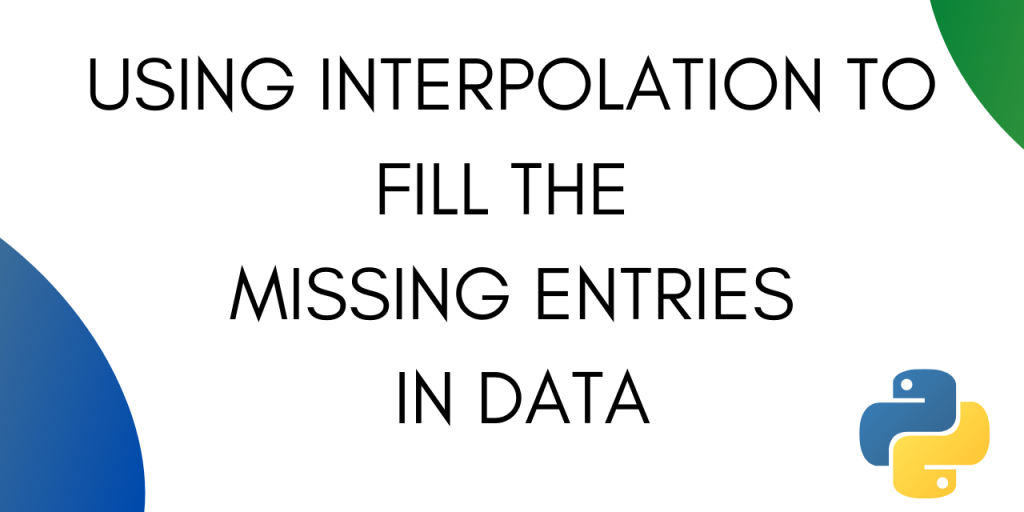# Using Interpolation To Fill Missing Entries in PythonInterpolation is a technique in Python with which you can estimate unknown data points between two known data points. It is commonly used to fill missing values in a table or a dataset using the already known values.

Interpolation is a technique that is also used in image processing. While expanding an image you can estimate the pixel value for a new pixel using the neighbouring pixels.

Financial analysts also use interpolation to predict the financial future using the know datapoints from the past.

In this tutorial, we will be looking at interpolation to fill missing values in a dataset.

Pandas Dataframe provides a .interpolate() method that you can use to fill the missing entries in your data.

Let’s create some dummy data and see how interpolation works.

## Using Interpolation for Missing Values in Series Data

Let’s create a Pandas series with a missing value.

```import pandas as pd
import numpy as np
a=pd.Series([0, 1, np.nan, 3,4,5,7])
```

### 1. Linear Interpolation

As you can see the value at the second index is nan. Interpolate the data with the following line of code:

```a.interpolate()
```

The output comes out as. :

```0    0.0
1    1.0
2    2.0
3    3.0
4    4.0
5    5.0
6    7.0
```

Pandas offers multiple methods of interpolation. Linear interpolation is the default method in case nothing is specified.

Let’s try another type of interpolation on the same data.

### 2. Polynomial interpolation

Polynomial interpolation requires you to specify an order. Let’s try interpolating with order 2.

```a.interpolate(method='polynomial', order=2)
```

The output comes out as :

```0    0.00000
1    1.00000
2    1.99537
3    3.00000
4    4.00000
5    5.00000
6    7.00000
```

If you give the order as 1 in polynomial interpolation then you get the same output as linear interpolation. This is because a polynomial of order 1 is linear.

```a.interpolate(method='polynomial', order=1)
```

Output :

```0    0.0
1    1.0
2    2.0
3    3.0
4    4.0
5    5.0
6    7.0
```

Interpolation through padding means copying the value just before a missing entry.

While using padding interpolation, you need to specify a limit. The limit is the maximum number of nans the method can fill consecutively.

Let’s see how it works in python.

```a.interpolate(method='pad', limit=2)
```

We get the output as :

```0    0.0
1    1.0
2    1.0
3    3.0
4    4.0
5    5.0
6    7.0
```

The missing entry is replaced by the same value as that of the entry before it.

We specified the limit as 2, let’s see what happens in case of three consecutive nans.

```a=pd.Series([0, 1, np.nan, np.nan, np.nan, 3,4,5,7])
```

The output comes as :

```0    0.0
1    1.0
2    1.0
3    1.0
4    NaN
5    3.0
6    4.0
7    5.0
8    7.0
```

The third nan is left untouched.

## Interpolation in Pandas DataFrames

We can also use interpolation to fill missing values in a pandas Dataframe.

Let’s create a dummy DataFrame and apply interpolation on it.

```s = pd.DataFrame([(0.0, np.nan, -2.0, 2.0), (np.nan, 2.0, np.nan, 1), (2.0, 5.0, np.nan, 9.0), (np.nan, 4.0, -3.0, 16.0)], columns=list('abcd'))
```

### 1. Linear Interpolation with Pandas Dataframe

To apply linear interpolation on the dataframe use the following line of code :

```s.interpolate()
```

Output :

Here the first value under the b column is still nan as there is no known data point before it for interpolation.

You can also interpolate individual columns of a dataframe.

```s['c'].interpolate()
```

Output :

```0   -2.000000
1   -2.333333
2   -2.666667
3   -3.000000
```

```s.interpolate(method='pad', limit=2)